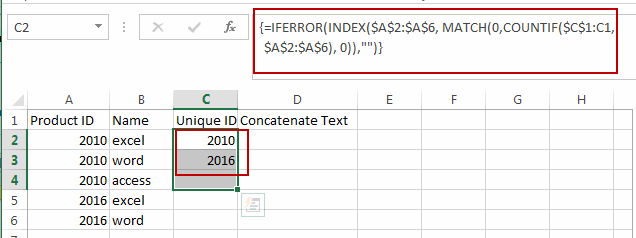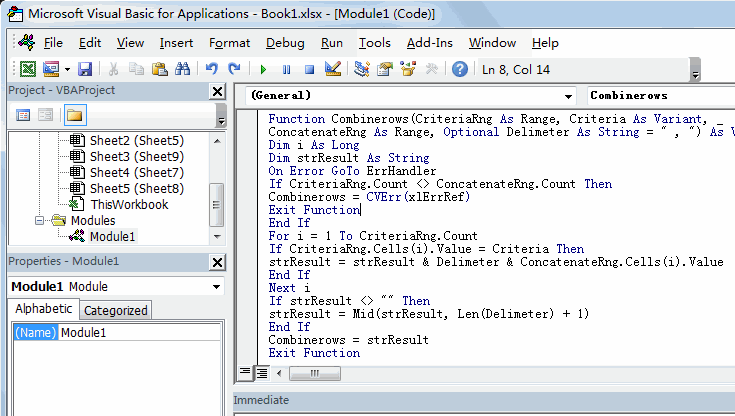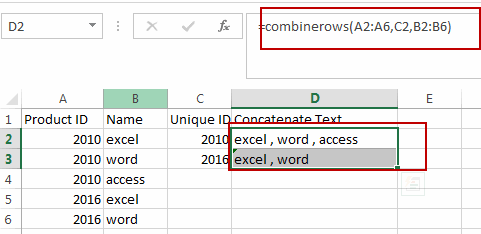# How to Concatenate Text based on unique values in Another Column in Excel

This post will guide you how to concatenate text values based on unique values in another column in Excel. How do I concatenate cells based on specific criteria in Excel.

## Concatenate Text Based on unique Values in Another Column

Assuming that you have a list of data in range A1:B6, in which contain product IDs and product Names. And you want to concatenate product names based on unique ID values (There are duplicated ID values in Column A), How to do it. You need to extract unique product IDs in another range, and then concatenating text values based on newly created range with a User Defined Function. Here are the steps:

#1 you can use an Excel Array formula based on the IFERROR function, the INDEX function, the MATCH function and the COUNTIF function to extract the unique product ID values.

`=IFERROR(INDEX(\$A\$2:\$A\$6, MATCH(0,COUNTIF(\$C\$1:C1, \$A\$2:\$A\$6), 0)),"")`

Type this formula into cell C2, and press Ctrl + Shift + Enter keys on your keyboard to change it as array formula.  And then drag the AutoFill Handle down to other cells until getting blank cells.Note: the range A2:A6 is a range which you want to extract unique values from it.  And the Cell C1 is the first cell of the column that you want to place the unique product ID values.

#2 open your excel workbook and then click on “Visual Basic” command under DEVELOPER Tab, or just press “ALT+F11” shortcut.#3 then the “Visual Basic Editor” window will appear.

#4 click “Insert” ->”Module” to create a new module.#5 paste the below VBA code (code from here) into the code window. Then clicking “Save” button.```Function Combinerows(CriteriaRng As Range, Criteria As Variant, _
ConcatenateRng As Range, Optional Delimeter As String = " , ") As Variant
Dim i As Long
Dim strResult As String
On Error GoTo ErrHandler
If CriteriaRng.Count <> ConcatenateRng.Count Then
Combinerows = CVErr(xlErrRef)
Exit Function
End If

For i = 1 To CriteriaRng.Count
If CriteriaRng.Cells(i).Value = Criteria Then
strResult = strResult & Delimeter & ConcatenateRng.Cells(i).Value
End If
Next i

If strResult <> "" Then
strResult = Mid(strResult, Len(Delimeter) + 1)
End If

Combinerows = strResult
Exit Function
ErrHandler:
Combinerows = CVErr(xlErrValue)
End Function```

#5 back to the current worksheet, then type the following formula in a blank cell, and then press Enter key.

=combinerows(A2:A6,C2,B2:B6)

#6 drag the AutoFill handle over other cells to  concatenate text based on unique product ID values.### Related Functions

• Excel IFERROR function
The Excel IFERROR function returns an alternate value you specify if a formula results in an error, or returns the result of the formula.The syntax of the IFERROR function is as below:= IFERROR (value, value_if_error)…
• Excel COUNTIF function
The Excel COUNTIF function will count the number of cells in a range that meet a given criteria. This function can be used to count the different kinds of cells with number, date, text values, blank, non-blanks, or containing specific characters.etc.= COUNTIF (range, criteria)…
• Excel INDEX function
The Excel INDEX function returns a value from a table based on the index (row number and column number)The INDEX function is a build-in function in Microsoft Excel and it is categorized as a Lookup and Reference Function.The syntax of the INDEX function is as below:= INDEX (array, row_num,[column_num])…
• Excel MATCH function
The Excel MATCH function search a value in an array and returns the position of that item.The syntax of the MATCH function is as below:= MATCH  (lookup_value, lookup_array, [match_type])….
Related Posts

How to Extract First Letter from Each Word in a Cell in Excel

This post will guide you how to extract first letter from each word in a given cell in Excel. How do I extract the first letter of each word in a range of names in your current worksheet in Microsoft ...

How to ignore Blank Cells while Concatenating cells in Excel

This post will guide you how to concatenate cells but ignore all blank cells in your worksheet in Excel. How do I concatenate cells but ignore blank cells with a formula in Excel. How to create a concatenate formula to ...

How to Calculate the Average Excluding the Smallest & Highest Numbers in Excel

Calculating the average for a batch of data is frequently used in our daily life. But for some cases like statistic the average score in a competition, or price analysis, we often calculate the average excluding the smallest and highest ...

How to Get the Maximum or Minimum Absolute Value in Excel

It is easy to find the maximum or minimum value in a batch of data in excel, but if this batch of numbers contains both positive and negative numbers, the maximum or minimum absolute value cannot be found out by ...

How to Count Only Numbers in Bold in a Range of Cells in Excel

This post will guide you how to Count only numbers with bold style in a range of cells in Excel. How do I Count on cells with bold font within a range of cells using User defined function in Excel ...

How to Sum Only Numbers in Bold in a Range of Cells in Excel

This post will guide you how to sum only numbers with bold style in a range of cells in Excel. How do I sum on cells with bold font within a range of cells using User defined function in Excel ...

How to Filter Formula in Excel

This post will guide you how to filter your data with an Formula in Excel. How do I filter cells with formulas through a User defined function in Excel 2013/2016. Filter Formula Assuming that you have a list of data ...

How to Count Cells by Font Color in Excel

This post will guide you how to count cells by font color in Excel. How do I Count the number of cells with specific cell Font color by using a User Defined function in Excel. Count Cells by Font Color ...

How to Highlight Cells Containing Formulas in Excel

This post will guide you how to highlight cells in which contain formulas using Conditional Formatting feature in Excel. How do I conditionally format a cell if it contains formula using a User defined function in combination with Conditional Formatting ...

How to Extract Number from Text String in Excel

This post will guide you how to extract number from a given test string in Excel. How do I extract all numbers from string using a formula in Excel. How to get all number from a given test string using ...

Sidebar